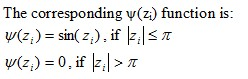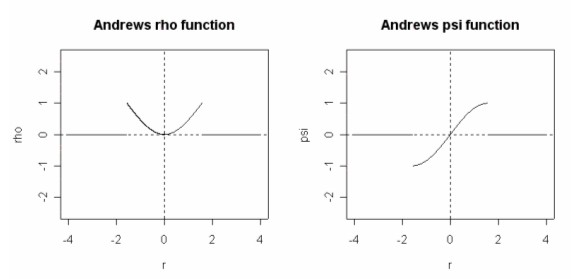# M-estimators

Statistical Analysis Techniques, Robust Estimators, Alternatives to OLS

The three main classes of robust estimators are M, L and R.  Robust estimators are resistant to outliers and when used in regression modelling, are robust to departures from the normality assumption.

M-estimators are a maximum likelihood type estimator.  M estimation involves minimizing the following: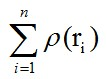Where ρ is some function with the following properties:
• ρ(r) ≥ 0 for all r and has a minimum at 0
• ρ(r) = ρ(-r) for all r
• ρ(r) increases as r increases from 0, but doesn’t get too large as r increases

For OLS:  ρ(r) = r2

Note that OLS doesn’t satisfy the third property, therefore it doesn’t count as a robust M-estimator.

In the case of a linear model, the function to minimise will be: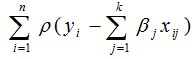Instead of minimising the function directly, it may be simpler to use the function’s first order conditions set to zero: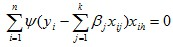where:If the ρ function can be differentiated, the M-estimator is said to be a ψ-type. Otherwise, the M-estimator is said to be a ρ-type.

### Lp subclass

Lp is a subclass of M estimators.  An Lp beta coefficient would be one that minimises the following: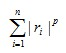Where 1≤ p ≤2.
If p=1, it is the equivalent of LAD and if p=2, it is the equivalent of OLS.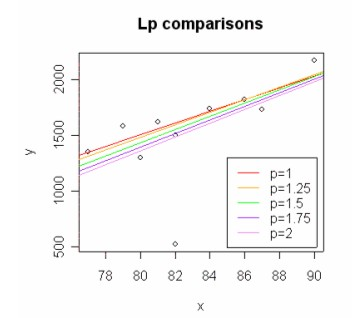The lower p is, the more robust the Lp will be to outliers.  The lower p is, the greater the number of iterations would be needed for the sum of |r|p to converge at the minimum.

### Tukey’s bisquare M-estimator

Tukey proposed an M-estimator that has the following ρ(zi) function:Where c is a constant and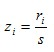, where s is the estimated scale parameter.

Tukey’s bisquare psi function leaves out any extreme outliers by giving them a zero weighting.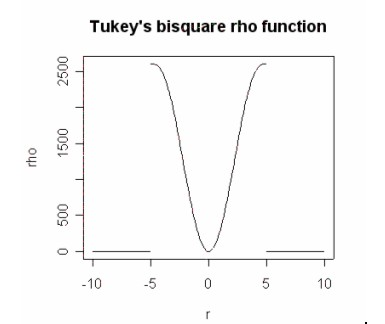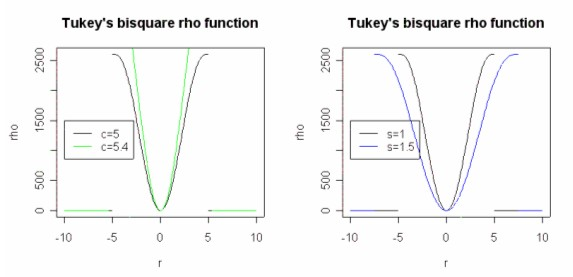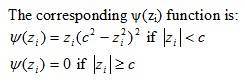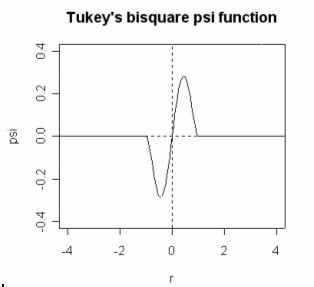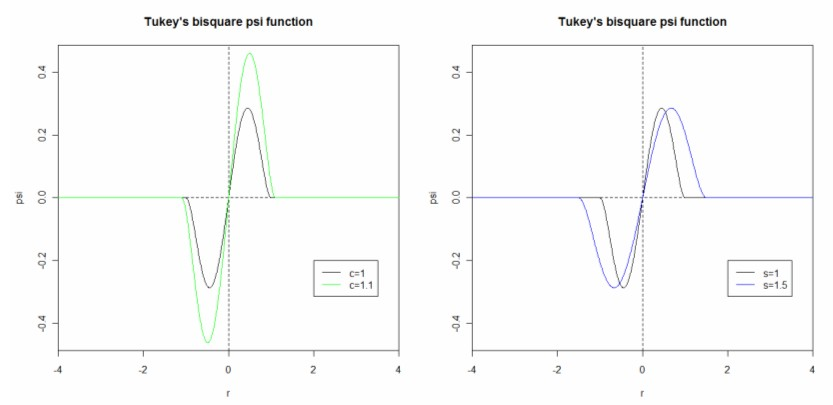### Huber's M-estimator

Huber proposed an M-estimator that has the following ρ(zi) function:Where c is a constant and, where s is the estimated scale parameter.

It essentially applies an LAD function to outliers and an OLS function to the other observations.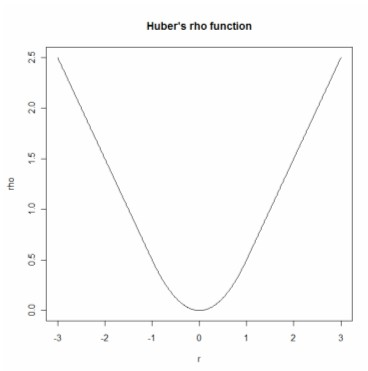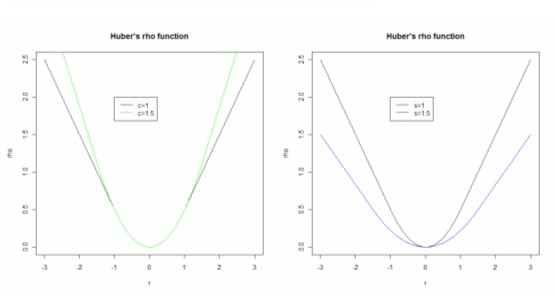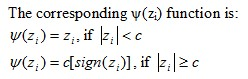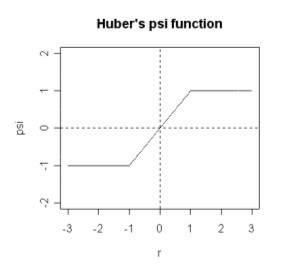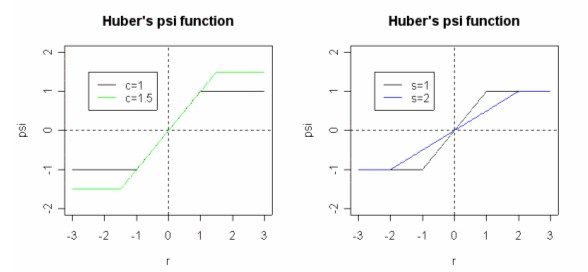### Andrews's M-estimator

Andrews (1974) proposed the following ρ(zi) function: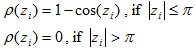Where, where s is the estimated scale parameter.